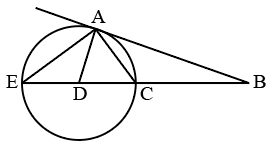Question 8

# AB is the tangent on the circle at point A. The line BC meets the circle at points C and E. Line AD bisects the angle EAC. If angle EAC = $$60^\circ$$ and angle BAC : angle ACB = 2: 5. Find angle ABC :Solution

Let us consider angle CAB=2x and angle ACB=5x

From Alternate segment theorem,

angle AEC = 2x

In triangle AEC, 60+180-5x+2x=180

3x=60

x=20

In triangle ABC, angle ABC = 180-7*20=40